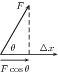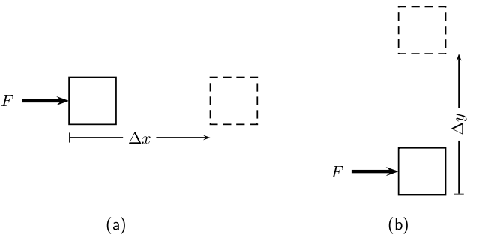# Work

 Page 1 / 3

## Introduction

Imagine a vendor carrying a basket of vegetables on her head. Is she doing any work? One would definitely say yes! However, in Physics she is not doing any work! Again, imagine a boy pushing against a wall? Is he doing any work? We can see that his muscles are contracting and expanding. He may even be sweating. But in Physics, he is not doing any work!

If the vendor is carrying a very heavy load for a long distance, we would say she has lot of energy. By this, we mean that she has a lot of stamina. If a car can travel very fast, we describe the car as powerful. So, there is a link between power and speed. However, power means something different in Physics. This chapter describes the links between work, energy and power and what these mean in Physics.

You will learn that work and energy are closely related. You shall see that the energy of an object is its capacity to do work and doing work is the process of transferring energy from one object or form to another. In other words,

• an object with lots of energy can do lots of work.
• when work is done, energy is lost by the object doing work and gained by the object on which the work is done.

Lifting objects or throwing them requires that you do work on them. Even making electricity flow requires that something do work. Something must have energy and transfer it through doing work to make things happen.

## Work

Work

When a force exerted on an object causes it to move, work is done on the object (except if the force and displacement are at right angles to each other).

This means that in order for work to be done, an object must be moved a distance $d$ by a force $F$ , such that there is some non-zero component of the force in the direction of the displacement. Work is calculated as:

$W=F·\Delta xcos\theta .$

where $F$ is the applied force, $\Delta x$ is the displacement of the object and $\theta$ is the angle between the applied force and the direction of motion.The force F causes the object to be displaced by Δ x at angle θ .

It is very important to note that for work to be done there must be a component of the applied force in the direction of motion. Forces perpendicular to the direction of motion do no work.

For example work is done on the object in [link] ,(a) The force F causes the object to be displaced by Δ x in the same direction as the force. θ = 0 ∘ and cos θ = 1 . Work is done in this situation. (b) A force F is applied to the object. The object is displaced by Δ y at right angles to the force. θ = 90 ∘ and cos θ = 0 . Work is not done in this situation.

## Investigation : is work done?

Decide whether on not work is done in the following situations. Remember that for work to be done a force must be applied in the direction of motion and there must be a displacement. Give reasons for your answer.

1. Max pushes against a wall and becomes tired.
2. A book falls off a table and free falls to the ground.
3. A rocket accelerates through space.
4. A waiter holds a tray full of meals above his head with one arm and carries it straight across the room at constant speed. (Careful! This is a tricky question.)

how does cell look like
What is impulse and how does it = to momentum
what is physics
what is a catanation ?
The ability of a few elements, most especially carbon, to yield chains and rings by forming covalent bonds with atoms of the same element.
Ofentse
different structural formula
different type of structural formula
Lucas
primary,secondary and tertiary
Snowy
how does the earth revolute
What is the momentum
The product of the object's mass and it's velocity
Mvula
electric field def
FREENCO
what is secondary alcohol?
Raymond
is when Carbon that is bonded with OH is also bonded to 2 carbons of the chain.
Gontse
Electric field is the region of space around a charged object where other charged objects will experience an electrostatic force ( coulomb forces) without coming into contact with the object that is generating the field.
Kagiso
Electric field is the region of space around a charged object where other charged objects will experience an electrostatic force ( coulomb forces) without coming into contact with the object that is generating the field.
Kagiso
how is ester formed
how is n ester formed
Aubrey
Alcohol reacts with a carboxylic acid
Texas
and the reaction is catalysed by sulphuric acid
Vhulenda
An ester is form when an alcohol reacts a carboxylic acid and sulphuric acid is used as a catalyst which therefore eliminates water.
Azola
an athlete with a mass of 70kg runs at a velocity of 45km . determine the athlete's momentum
Is that a velocity or something else
msawenkosi
45km/h i guess
Texas
Change to m/s
Texas
45km/h = 12.5 m/s p=mv =70×12.5 =875 kg.m/s
Thato
what are the measures of the rates of reaction
Volume Concentration Temperature Pressure Surface Area
Thato
the principle of superposition of waves
what is work
is this a group chat
Hey can y'all define newton's 2nd law
mthebzification
If a resultant force act on an object...the object will accelerate in the direction of a resultant force,the acceleration of the object is directly proportional to the net force and inversely proportional to the mass of the object
mosa
how do you calculate tension force
Bulumko
use the formula Fnet=ma if there is tension connecting two objects
Sboniso
to calculate Tension, usually calculate acceleration first Draw separate free body diagrams for each body. Apply Fnet = ma to calculate Tension
Kevin
Hi people
Paul
when a resultant force acting on an object the object will accelerate in the direction of a force at an acceleration directly proportional to the force and invesly proportional to the mass of the object.
Raymond
Hey people
Kaytee
how does temperature affect the equilibrium position
an increased temperature increases the average kinetic energy thus in turn increases the number of effective collisions........
Lwando
so...which reaction is favored between endothermic and exothermic .when temperature is increased..?
Blessing
exothermic reaction because energy is realised to the surroundings as heat and light energy ....graphical so much energy is realised as reactants to form product and because temperature is high rate of reaction is fast which means there is a successful collision
Code
INTEMENDO - INCREASE IN TEMPERATURE FAVOURS ENDOTHERMIC DETEMEXO - DECREASE IN TEMPERATURE FAVOURS EXOTHERMIC
Thato
an object will continue in a state of rest unless it is acted upon an unbalanced force
Newton's Law 1
Code
First Newton's Law
Azola
Newton's first law
Surprise
newton first law
Thinavhuyo
Newton's first law
BlessingBy Subramanian DivyaByBy MldelatteByBy Edward BitonBy